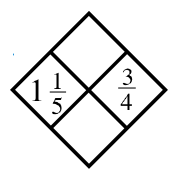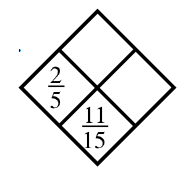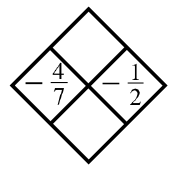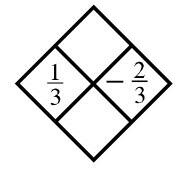### Home > CC2MN > Chapter 9 > Lesson 9.3.1 > Problem9-97

9-97.

Copy and complete each of the Diamond Problems below. The pattern used in the Diamond Problems is shown at right.1.Converting the mixed number into a fraction greater than one may prove to be helpful.

$\left(\frac{6}{5}\right)\left(\frac{3}{4}\right) = ?$

$\frac{6}{5} + \frac{3}{4} = ?$

Top: $\frac{9}{10}$
Bottom: $\frac{39}{20}$

1.$\frac{2}{5} + ? = \frac{11}{15}$

Top: $\frac{2}{15}$
Right: $\frac{1}{3}$

1.Use the method presented in part (a).

1.Use the method presented in part (a).